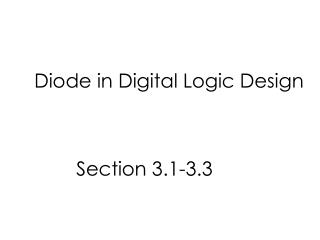DownloadDownload PresentationDiode in Digital Logic Design

# Diode in Digital Logic Design

Download Presentation## Diode in Digital Logic Design

- - - - - - - - - - - - - - - - - - - - - - - - - - - E N D - - - - - - - - - - - - - - - - - - - - - - - - - - -
##### Presentation Transcript

1. Diode in Digital Logic Design Section 3.1-3.3

2. Schedule

3. Outline • Review • Diode Model • Applications of Diodes in Digital Logic • OR2 • AND2

4. Different ways of Crossing PN Junction Diffusion Diffusion np=ni2 Drift Drift Majority carriers cross the pn junction via diffusion (because you have the gradient) Minority carriers cross the pn junction via drift( because you have the E, not the gradient)

5. Reverse Biased Diode Reverse: Connect the + terminal to the n side. Depletion region widens. Therefore, stronger E. Minority carrier to cross the PN junction easily through drift. Current is composed mostly of drift current contributed by minority carriers. np to the left and pn to the right. Current from n side to p side, the current is negative. E

6. Forward Biased Diode Depletion region shrinks due to charges from the battery. The electric field is weaker. Majority carrier can cross via diffusion; Greater diffusion current. Current flows from P side to N side

7. c02f31 IS=Reverse Saturation=leakage current

8. Diode Models (Ideal model) (Exponential model) (Constant voltage model)

9. Choosing a Diode Model Use the ideal model to develop a quick, rough understanding of a circuit. If the ideal model is not adequate, uses the constant voltage model, which is sufficient for most cases. Occasionally, we will use the exponential model

10. Ideal Model of a Diode (exponential model) (ideal model) An ideal diode will turn on even for the slightest forward bias voltage. (VD≥0) An ideal diode will turn off even for the slightest reverse bias voltage. (VD<0)

11. c03f03 Behavior of Ideal Diode Ideal diode: Vanode>Vcathode: Diode is on Vanode<Vcathode: Diode is off An ideal current experieincing Vanode=Vcathode, carries no current

12. c03f05 I/V Characteristics A short--can’t get a V to develop across a diode. An Open—can’t get a current to flow. A diode Vanode>Vcathode: Diode is on Vanode<Vcathode: Diode is off An ideal current experieincing Vanode=Vcathode, carries no current In practice, consider a slightly positive or negative voltage to determine the response of a diode.

13. c03f08 Example 1: An OR Gate Realized By Diodes “0”=0 V “0”=0 V Assume that “1”=3 V “0”=0 V Assume “ideal” diode

14. c03f08 Exercise 1: An OR Gate Realized By Diodes “1”=3V “0”=3 V Assume that “1”=3 V “0”=0 V Assume “ideal” diode What is Vout?

15. c03f08 Exercise 2: An OR Gate Realized By Diodes “1”=3V “1”=3 V Assume that “1”=3 V “0”=0 V Assume “ideal” diode What is Vout?

16. Analysis of an OR Gate Logic 1=3 V Logic 0=0V • Observations: • If D1 is on, VA=VOUT and VOUT=“1” • If D2 is on, VB=VOUT and VOUT=“1”. • VOUT is 0 if and only if D1 and D2 are “0” • This is an OR gate.

17. Cadence Simulation of an OR Gate VA=3 V VB=3 V VOUT=2.459 V≈3V

18. Cadence Simulation of an OR Gate VA=3 V VB=0 V VOUT=2.424 V≈3V

19. Cadence Simulation of an OR Gate VA=0 V VB=0 V VOUT=0 V

20. c02f33 Constant Voltage Model If VD is less than VD, On, the diode behaves like an open circuit. The diode will behave like an open circuit for VD=VD,on

21. Cadence Simulation of an OR Gate VA=3 V VB=0 V VOUT=2.424 V Constant voltage model: 3V-0.6V=2.4 V If we assume a turn on voltage of 0.6 V, we are not off by too much.

22. Grid Control

23. Export Image Option File→Export Image

24. In Class Exercise What kind of gate is this? Please assume ideal diode model.

25. Cadence Simulation of an AND Gate VA=3 V VB=3 V VOUT=3 V

26. In Class Exercise Assume that VA=“1”=3V, VB=“0”=0V Please assume constant voltage model. What is the output voltage?

27. Cadence Simulation of an AND Gate VA=3 V VB=0 V VOUT=0.575 V